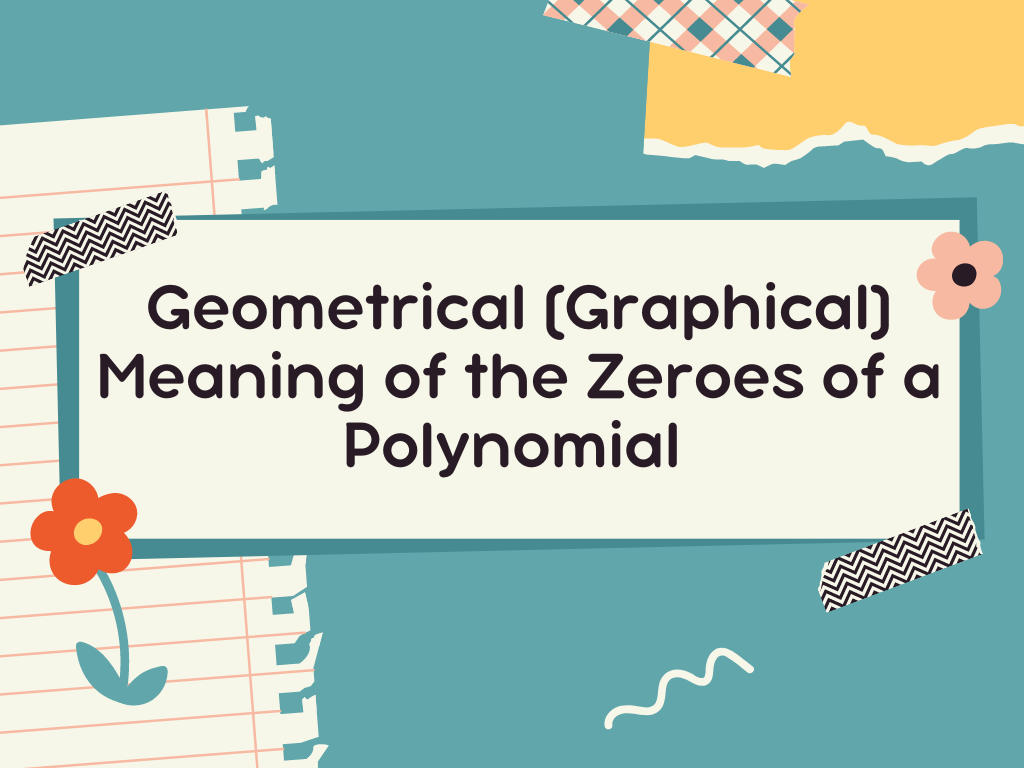## Geometrical (Graphical) Meaning of the Zeroes of a Polynomial Class 10th

Introduction We know that if a polynomial is p(x) and R is it’s zero then p(R) = 0. It means the zeroes of every polynomial satisfy it. Let’s understand this with the help of geometrical or graphical representation. In class 10th, We shall study the geometrical representation of linear polynomials, quadratic polynomials, and cubic polynomials, …